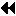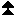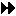## 10.2.5. Additional Support for Fortran Numeric Intrinsic TypesUp: Fortran Support Next: Parameterized Datatypes with Specified Precision and Exponent Range Previous: No Type Mismatch Problems for Subroutines with Choice Arguments

The routines in this section are part of Extended Fortran Support described in Section Extended Fortran Support .

MPI-1 provides a small number of named datatypes that correspond to named intrinsic types supported by C and Fortran. These include MPI_INTEGER, MPI_REAL, MPI_INT, MPI_DOUBLE, etc., as well as the optional types MPI_REAL4, MPI_REAL8, etc. There is a one-to-one correspondence between language declarations and MPI types.

Fortran (starting with Fortran 90) provides so-called KIND-parameterized types. These types are declared using an intrinsic type (one of INTEGER, REAL, COMPLEX, LOGICAL and CHARACTER) with an optional integer KIND parameter that selects from among one or more variants. The specific meaning of different KIND values themselves are implementation dependent and not specified by the language. Fortran provides the KIND selection functions selected_real_kind for REAL and COMPLEX types, and selected_int_kind for INTEGER types that allow users to declare variables with a minimum precision or number of digits. These functions provide a portable way to declare KIND-parameterized REAL, COMPLEX and INTEGER variables in Fortran. This scheme is backward compatible with Fortran 77. REAL and INTEGER Fortran variables have a default KIND if none is specified. Fortran DOUBLE PRECISION variables are of intrinsic type REAL with a non-default KIND. The following two declarations are equivalent:

```double precision x
real(KIND(0.0d0)) x
```
MPI provides two orthogonal methods to communicate using numeric intrinsic types. The first method can be used when variables have been declared in a portable way --- using default KIND or using KIND parameters obtained with the selected_int_kind or selected_real_kind functions. With this method, MPI automatically selects the correct data size (e.g., 4 or 8 bytes) and provides representation conversion in heterogeneous environments. The second method gives the user complete control over communication by exposing machine representations.Up: Fortran Support Next: Parameterized Datatypes with Specified Precision and Exponent Range Previous: No Type Mismatch Problems for Subroutines with Choice Arguments

### 10.2.5.1. Parameterized Datatypes with Specified Precision and Exponent RangeUp: Additional Support for Fortran Numeric Intrinsic Types Next: Support for Size-specific MPI Datatypes Previous: Additional Support for Fortran Numeric Intrinsic Types

MPI-1 provides named datatypes corresponding to standard Fortran 77 numeric types --- MPI_INTEGER, MPI_COMPLEX, MPI_REAL, MPI_DOUBLE_PRECISION and MPI_DOUBLE_COMPLEX. MPI automatically selects the correct data size and provides representation conversion in heterogeneous environments. The mechanism described in this section extends this MPI-1 model to support portable parameterized numeric types.

The model for supporting portable parameterized types is as follows. Real variables are declared (perhaps indirectly) using selected_real_kind(p, r) to determine the KIND parameter, where p is decimal digits of precision and r is an exponent range. Implicitly MPI maintains a two-dimensional array of predefined MPI datatypes D(p, r). D(p, r) is defined for each value of (p, r) supported by the compiler, including pairs for which one value is unspecified. Attempting to access an element of the array with an index (p, r) not supported by the compiler is erroneous. MPI implicitly maintains a similar array of COMPLEX datatypes. For integers, there is a similar implicit array related to selected_int_kind and indexed by the requested number of digits r. Note that the predefined datatypes contained in these implicit arrays are not the same as the named MPI datatypes MPI_REAL, etc., but a new set.

The above description is for explanatory purposes only. It is not expected that implementations will have such internal arrays. ( End of advice to implementors.)

selected_real_kind() maps a large number of (p,r) pairs to a much smaller number of KIND parameters supported by the compiler. KIND parameters are not specified by the language and are not portable. From the language point of view intrinsic types of the same base type and KIND parameter are of the same type. In order to allow interoperability in a heterogeneous environment, MPI is more stringent. The corresponding MPI datatypes match if and only if they have the same (p,r) value ( REAL and COMPLEX) or r value ( INTEGER). Thus MPI has many more datatypes than there are fundamental language types. ( End of advice to users.)

MPI_TYPE_CREATE_F90_REAL(p, r, newtype)
[ IN p] precision, in decimal digits (integer)
[ IN r] decimal exponent range (integer)
[ OUT newtype] the requested MPI datatype (handle)
int MPI_Type_create_f90_real(int p, int r, MPI_Datatype *newtype)
MPI_TYPE_CREATE_F90_REAL(P, R, NEWTYPE, IERROR)
INTEGER P, R, NEWTYPE, IERROR
static MPI::Datatype MPI::Datatype::Create_f90_real(int p, int r)

This function returns a predefined MPI datatype that matches a REAL variable of KIND selected_real_kind(p, r). In the model described above it returns a handle for the element D(p, r). Either p or r may be omitted from calls to selected_real_kind(p, r) (but not both). Analogously, either p or r may be set to MPI_UNDEFINED. In communication, an MPI datatype A returned by MPI_TYPE_CREATE_F90_REAL matches a datatype B if and only if B was returned by MPI_TYPE_CREATE_F90_REAL called with the same values for p and r or B is a duplicate of such a datatype. Restrictions on using the returned datatype with the ``external32'' data representation are given.

It is erroneous to supply values for p and r not supported by the compiler.

MPI_TYPE_CREATE_F90_COMPLEX(p, r, newtype)
[ IN p] precision, in decimal digits (integer)
[ IN r] decimal exponent range (integer)
[ OUT newtype] the requested MPI datatype (handle)

int MPI_Type_create_f90_complex(int p, int r, MPI_Datatype *newtype)
MPI_TYPE_CREATE_F90_COMPLEX(P, R, NEWTYPE, IERROR)
INTEGER P, R, NEWTYPE, IERROR
static MPI::Datatype MPI::Datatype::Create_f90_complex(int p, int r)

This function returns a predefined MPI datatype that matches a COMPLEX variable of KIND selected_real_kind(p, r). Either p or r may be omitted from calls to selected_real_kind(p, r) (but not both). Analogously, either p or r may be set to MPI_UNDEFINED. Matching rules for datatypes created by this function are analogous to the matching rules for datatypes created by MPI_TYPE_CREATE_F90_REAL. Restrictions on using the returned datatype with the ``external32'' data representation are given.

It is erroneous to supply values for p and r not supported by the compiler.

MPI_TYPE_CREATE_F90_INTEGER(r, newtype)
[ IN r] decimal exponent range, i.e., number of decimal digits (integer)
[ OUT newtype] the requested MPI datatype (handle)
int MPI_Type_create_f90_integer(int r, MPI_Datatype *newtype)
MPI_TYPE_CREATE_F90_INTEGER(R, NEWTYPE, IERROR)
INTEGER R, NEWTYPE, IERROR
static MPI::Datatype MPI::Datatype::Create_f90_integer(int r)

This function returns a predefined MPI datatype that matches a INTEGER variable of KIND selected_int_kind(r). Matching rules for datatypes created by this function are analogous to the matching rules for datatypes created by MPI_TYPE_CREATE_F90_REAL. Restrictions on using the returned datatype with the ``external32'' data representation are given.

It is erroneous to supply a value for r that is not supported by the compiler.

Example:

```integer       longtype, quadtype
integer, parameter :: long = selected_int_kind(15)
integer(long) ii(10)
real(selected_real_kind(30)) x(10)
call MPI_TYPE_CREATE_F90_INTEGER(15, longtype, ierror)
...

call MPI_SEND(ii, 10, longtype, ...)
```

The datatypes returned by the above functions are predefined datatypes. They cannot be freed; they do not need to be committed; they can be used with predefined reduction operations. There are two situations in which they behave differently syntactically, but not semantically, from the MPI named predefined datatypes.

1. MPI_TYPE_GET_ENVELOPE returns special combiners that allow a program to retrieve the values of p and r.
2. Because the datatypes are not named, they cannot be used as compile-time initializers or otherwise accessed before a call to one of the MPI_TYPE_CREATE_F90_ routines.
If a variable was declared specifying a non-default KIND value that was not obtained with selected_real_kind() or selected_int_kind(), the only way to obtain a matching MPI datatype is to use the size-based mechanism described in the next section.

( End of advice to users.)

[] Rationale.

The MPI_TYPE_CREATE_F90_REAL/COMPLEX/INTEGER interface needs as input the original range and precision values to be able to define useful and compiler-independent external (Section External Data Representation: ``external32'' ) or user-defined (Section User-Defined Data Representations ) data representations, and in order to be able to perform automatic and efficient data conversions in a heterogeneous environment. ( End of rationale.)
We now specify how the datatypes described in this section behave when used with the ``external32'' external data representation described in Section External Data Representation: ``external32'' .

The external32 representation specifies data formats for integer and floating point values. Integer values are represented in two's complement big-endian format. Floating point values are represented by one of three IEEE formats. These are the IEEE ``Single,'' ``Double'' and ``Double Extended'' formats, requiring 4, 8 and 16 bytes of storage, respectively. For the IEEE ``Double Extended'' formats, MPI specifies a Format Width of 16 bytes, with 15 exponent bits, bias = +10383, 112 fraction bits, and an encoding analogous to the ``Double'' format.

The external32 representations of the datatypes returned by MPI_TYPE_CREATE_F90_REAL/COMPLEX/INTEGER are given by the following rules.
For MPI_TYPE_CREATE_F90_REAL:

```if      (p > 33) or (r > 4931) then  external32 representation
is undefined
else if (p > 15) or (r >  307) then  external32_size = 16
else if (p >  6) or (r >   37) then  external32_size =  8
else                                 external32_size =  4
```
For MPI_TYPE_CREATE_F90_COMPLEX: twice the size as for MPI_TYPE_CREATE_F90_REAL.
For MPI_TYPE_CREATE_F90_INTEGER:
```if      (r > 38) then  external32 representation is undefined
else if (r > 18) then  external32_size =  16
else if (r >  9) then  external32_size =  8
else if (r >  4) then  external32_size =  4
else if (r >  2) then  external32_size =  2
else                   external32_size =  1
```
If the external32 representation of a datatype is undefined, the result of using the datatype directly or indirectly (i.e., as part of another datatype or through a duplicated datatype) in operations that require the external32 representation is undefined. These operations include MPI_PACK_EXTERNAL, MPI_UNPACK_EXTERNAL and many MPI_FILE functions, when the ``external32'' data representation is used. The ranges for which the external32 representation is undefined are reserved for future standardization.Up: Additional Support for Fortran Numeric Intrinsic Types Next: Support for Size-specific MPI Datatypes Previous: Additional Support for Fortran Numeric Intrinsic Types

### 10.2.5.2. Support for Size-specific MPI DatatypesUp: Additional Support for Fortran Numeric Intrinsic Types Next: Communication With Size-specific Types Previous: Parameterized Datatypes with Specified Precision and Exponent Range

MPI-1 provides named datatypes corresponding to optional Fortran 77 numeric types that contain explicit byte lengths --- MPI_REAL4, MPI_INTEGER8, etc. This section describes a mechanism that generalizes this model to support all Fortran numeric intrinsic types.

We assume that for each typeclass (integer, real, complex) and each word size there is a unique machine representation. For every pair ( typeclass, n) supported by a compiler, MPI must provide a named size-specific datatype. The name of this datatype is of the form MPI_<TYPE>n in C and Fortran and of the form MPI::<TYPE>n in C++ where <TYPE> is one of REAL, INTEGER and COMPLEX, and n is the length in bytes of the machine representation. This datatype locally matches all variables of type ( typeclass, n). The list of names for such types includes:

```MPI_REAL4
MPI_REAL8
MPI_REAL16
MPI_COMPLEX8
MPI_COMPLEX16
MPI_COMPLEX32
MPI_INTEGER1
MPI_INTEGER2
MPI_INTEGER4
MPI_INTEGER8
MPI_INTEGER16
```
In MPI-1 these datatypes are all optional and correspond to the optional, nonstandard declarations supported by many Fortran compilers. In MPI-2, one datatype is required for each representation supported by the compiler. To be backward compatible with the interpretation of these types in MPI-1, we assume that the nonstandard declarations REAL*n, INTEGER*n, always create a variable whose representation is of size n. All these datatypes are predefined.

The following functions allow a user to obtain a size-specific MPI datatype for any intrinsic Fortran type.

MPI_SIZEOF(x, size)
[ IN x] a Fortran variable of numeric intrinsic type (choice)
[ OUT size] size of machine representation of that type (integer)

MPI_SIZEOF(X, SIZE, IERROR)
<type> X
INTEGER SIZE, IERROR

This function returns the size in bytes of the machine representation of the given variable. It is a generic Fortran routine and has a Fortran binding only.

This function is similar to the C and C++ sizeof operator but behaves slightly differently. If given an array argument, it returns the size of the base element, not the size of the whole array. ( End of advice to users.)

[] Rationale.

This function is not available in other languages because it would not be useful. ( End of rationale.)
MPI_TYPE_MATCH_SIZE(typeclass, size, type)
[ IN typeclass] generic type specifier (integer)
[ IN size] size, in bytes, of representation (integer)
[ OUT type] datatype with correct type, size (handle)

int MPI_Type_match_size(int typeclass, int size, MPI_Datatype *type)
MPI_TYPE_MATCH_SIZE(TYPECLASS, SIZE, TYPE, IERROR)
INTEGER TYPECLASS, SIZE, TYPE, IERROR
static MPI::Datatype MPI::Datatype::Match_size(int typeclass, int size)

typeclass is one of MPI_TYPECLASS_REAL, MPI_TYPECLASS_INTEGER and MPI_TYPECLASS_COMPLEX, corresponding to the desired typeclass. The function returns an MPI datatype matching a local variable of type ( typeclass, size).

This function returns a reference (handle) to one of the predefined named datatypes, not a duplicate. This type cannot be freed. MPI_TYPE_MATCH_SIZE can be used to obtain a size-specific type that matches a Fortran numeric intrinsic type by first calling MPI_SIZEOF in order to compute the variable size, and then calling MPI_TYPE_MATCH_SIZE to find a suitable datatype. In C and C++, one can use the C function sizeof(), instead of MPI_SIZEOF. In addition, for variables of default kind the variable's size can be computed by a call to MPI_TYPE_GET_EXTENT, if the typeclass is known. It is erroneous to specify a size not supported by the compiler.

[] Rationale.

This is a convenience function. Without it, it can be tedious to find the correct named type. See note to implementors below. ( End of rationale.)

This function could be implemented as a series of tests.

```int MPI_Type_match_size(int typeclass, int size, MPI_Datatype *rtype)
{
switch(typeclass) {
case MPI_TYPECLASS_REAL: switch(size) {
case 4: *rtype = MPI_REAL4; return MPI_SUCCESS;
case 8: *rtype = MPI_REAL8; return MPI_SUCCESS;
default: error(...);
}
case MPI_TYPECLASS_INTEGER: switch(size) {
case 4: *rtype = MPI_INTEGER4; return MPI_SUCCESS;
case 8: *rtype = MPI_INTEGER8; return MPI_SUCCESS;
default: error(...);       }
... etc ...
}
}
```
( End of advice to implementors.)Up: Additional Support for Fortran Numeric Intrinsic Types Next: Communication With Size-specific Types Previous: Parameterized Datatypes with Specified Precision and Exponent Range

### 10.2.5.3. Communication With Size-specific TypesUp: Additional Support for Fortran Numeric Intrinsic Types Next: Bibliography Previous: Support for Size-specific MPI Datatypes

The usual type matching rules apply to size-specific datatypes: a value sent with datatype MPI_<TYPE>n can be received with this same datatype on another process. Most modern computers use 2's complement for integers and IEEE format for floating point. Thus, communication using these size-specific datatypes will not entail loss of precision or truncation errors.

Care is required when communicating in a heterogeneous environment. Consider the following code:

```real(selected_real_kind(5)) x(100)
call MPI_SIZEOF(x, size, ierror)
call MPI_TYPE_MATCH_SIZE(MPI_TYPECLASS_REAL, size, xtype, ierror)
if (myrank .eq. 0) then
... initialize x ...
call MPI_SEND(x, xtype, 100, 1, ...)
else if (myrank .eq. 1) then
call MPI_RECV(x, xtype, 100, 0, ...)
endif
```
This may not work in a heterogeneous environment if the value of size is not the same on process 1 and process 0. There should be no problem in a homogeneous environment. To communicate in a heterogeneous environment, there are at least four options. The first is to declare variables of default type and use the MPI datatypes for these types, e.g., declare a variable of type REAL and use MPI_REAL. The second is to use selected_real_kind or selected_int_kind and with the functions of the previous section. The third is to declare a variable that is known to be the same size on all architectures (e.g., selected_real_kind(12) on almost all compilers will result in an 8-byte representation). The fourth is to carefully check representation size before communication. This may require explicit conversion to a variable of size that can be communicated and handshaking between sender and receiver to agree on a size.

Note finally that using the ``external32'' representation for I/O requires explicit attention to the representation sizes. Consider the following code:

```real(selected_real_kind(5)) x(100)
call MPI_SIZEOF(x, size, ierror)
call MPI_TYPE_MATCH_SIZE(MPI_TYPECLASS_REAL, size, xtype, ierror)

if (myrank .eq. 0) then
call MPI_FILE_OPEN(MPI_COMM_SELF, 'foo',                &
MPI_MODE_CREATE+MPI_MODE_WRONLY,     &
MPI_INFO_NULL, fh, ierror)
call MPI_FILE_SET_VIEW(fh, 0, xtype, xtype, 'external32',  &
MPI_INFO_NULL, ierror)
call MPI_FILE_WRITE(fh, x, 100, xtype, status, ierror)
call MPI_FILE_CLOSE(fh, ierror)
endif

call MPI_BARRIER(MPI_COMM_WORLD, ierror)

if (myrank .eq. 1) then
call MPI_FILE_OPEN(MPI_COMM_SELF, 'foo', MPI_MODE_RDONLY,  &
MPI_INFO_NULL, fh, ierror)
call MPI_FILE_SET_VIEW(fh, 0, xtype, xtype, 'external32',  &
MPI_INFO_NULL, ierror)
call MPI_FILE_WRITE(fh, x, 100, xtype, status, ierror)
call MPI_FILE_CLOSE(fh, ierror)
endif

```
If processes 0 and 1 are on different machines, this code may not work as expected if the size is different on the two machines. ( End of advice to users.)Up: Additional Support for Fortran Numeric Intrinsic Types Next: Bibliography Previous: Support for Size-specific MPI Datatypes